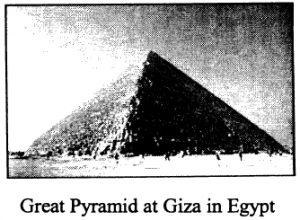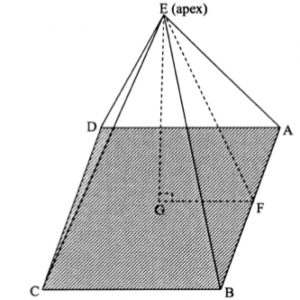The Great Pyramid at Giza in Egypt was built around 2500 BC. The pyramid has a square base ABCD with sides 232.6 metres long. The distance from each corner of the base to the apex E was originally 221.2 metres.You may find it helpful to make a square based pyramid with paper sticks and cut out a paper triangle like triangle EGF to fit inside.

Draw a diagram of the pyramid to show two right angled triangles from which you can write down trigonometric equations to answer the following questions.1. If F is a point on AB such that EF is perpendicular to AB and G is vertically below the apex E , what can you say about triangles EFA and EGF?
2. Calculate the size of the angle at the apex of a face of the pyramid (for example angle AEB). Can you find this by two different methods?
3. Calculate the angle each face makes with the base  (for example angle EFG)